## 概率统计17——点估计和连续性修正

概率（probabilty）和统计（statistics）是两个相近的概念，其实研究的问题刚好相反。

概率是使用一个已知参数的模型去预测这个模型所产生的结果，并研究结果的相关数字特征，比如期望、方差等。假设现在已知一个射击运动员的得分服从均值为8.2，方差为1.5的正态分布，就可以对这名运动员下一次射击的得分情况有个大致的预估。

统计与概率正好相反，是先有一堆数据，然后利用这些数据推测出模型和模型的参数。现在来了一名陌生的运动员，我们对他一无所知，不过他宣称自己是一名优秀的职业运动员。在进行了一系列射击测试后，教练组收集到了一批这名运动员的成绩，通过观察数据，认为他的成绩符合正态分布（也就是确定了模型），之后再进一步通过数据推测模型参数的具体值。对于正态分布来说，参数是均值和方差。

这样看来，我们碰到的大多数问题都是统计问题，虽然概率是随机事件的客观规律，但遗憾的是，这个规律总是作为未知量出现的，作为补偿，我们拥有一系列数据样本，虽然这些样本可能远小于整体，但仍然可以以点带面，根据这些样本对整体参数进行估计，得出关于整体概率分布的近似值。这个根据样本对总体参数进行估计的过程就是参数估计。根据参数性质不同，可分为点估计和区间估计。

点估计就是用样本统计量的某一具体数值直接推断未知的总体参数，得到的是一个具体的参数值。我们之前讲过的矩估计、最大似然估计、贝叶斯估计都是点估计。

我们以矩估计为例，重新看看怎样由样本估计总体。

# 总体数量和样本数量

我们经常用n表示总体的数量，究竟什么才算总体呢？

总体总是给人以“多”的概念，但事实并非如此，不同问题的“总体”数量可能相差很远。比如一个啤酒厂一年生产的罐装啤酒是1000万，一个班级的学生数是60人，无论是1000万还是60，都是总体。用n表示总体的数量。

既然是用样本估计总体，当然少不了抽样，关于抽样可参考：​数据分析(4)——闲话抽样 | 看似公平的随机抽样是否真的公平？。 用m表示样本的数量。

# 估计总体的均值

医生的判断很大程度依赖于血液检测的结果。从抽血结束到取得报告单需要一段时间，这段时间并不确定，也许运气较好，十几分钟就拿到了，也可能等上一小时。现在得到了一组样本，X = {x1, x2, ……, xm}，其中每个数据代表了一名患者取得报告单前的等待时间。我们的目标是根据这组样本对整体的平均等待时间做一个估计。

计算方式很简单，只需要计算样本的均值就可以了：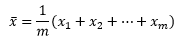我们认为样本的分布与整体的分布相似，这个均值是根据目前已知的数据对总体的最佳近似描述。这种用样本均值估计总体均值的方法称为矩估计，估计的结果是总体均值的点估计量。

下面的代码展现了整体分布和抽样分布的关系：

import numpy as np
import matplotlib.pyplot as plt
from scipy import stats

fig = plt.figure(figsize=(10, 5))

mu, sigma_square = 30, 5 # 均值和方差
sigma = sigma_square ** 0.5 # 标准差
xs = np.arange(15, 45, 0.5)
ys = stats.norm.pdf(xs, mu, sigma)
ax.plot(xs, ys, label='密度曲线')
ax.vlines(mu, 0, 0.2, linestyles='--', colors='r', label='均值')
ax.legend(loc='upper right')
ax.set_xlabel('X')
ax.set_ylabel('pdf')
ax.set_title('X~N($\mu$, $\sigma^2$), $\mu$={0}, $\sigma^2$={1}'.format(mu, sigma_square))

for i in [1, 2, 3]:
m = 10 ** i # 样本数量
np.random.seed(m)
X = stats.norm.rvs(loc=mu, scale=sigma, size=m) # 生成m个符合正态分布的随机变量
X = np.trunc(X) # 对数据取整
mu_x = X.mean() # 样本均值
ax = fig.add_subplot(2, 2, i + 1)
ax.hist(X, bins=40)
ax.set_xlabel('X')
ax.set_ylabel('频度')
ax.set_title('m={0},样本均值={1}'.format(m, mu_x))

plt.rcParams['font.sans-serif'] = ['SimHei']  # 用来正常显示中文标签
# plt.rcParams['axes.unicode_minus'] = False  # 解决中文下的坐标轴负号显示问题
plt.show()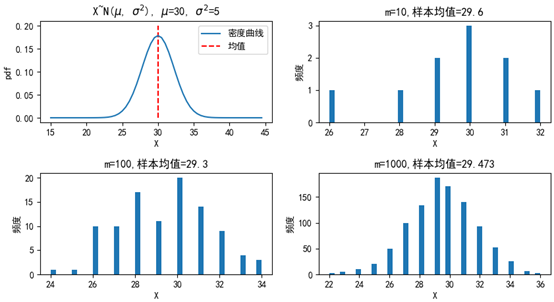可以看到，抽取的样本越多，样本的分布越接近整体的分布。这里有问题的是均值的符号，在各种资料中一会是μ，一会是戴帽子的μ，一会是x拔，到底用哪个？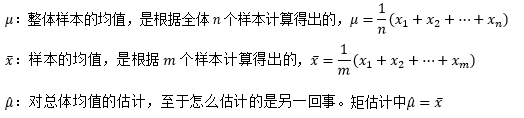过去我们一直说某些问题符合X~N(μ, σ2)的分布，这里μ是总体均值；在最大似然估计中得出的结果用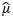表示，说明这是通过样本对整体均值的一个点估计量。

# 估计总体的方差

假设我们已经预先计算出了均值的点估计量，是否可以像计算总体方差一样计算样本方差呢？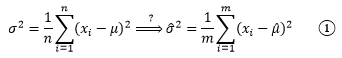从上面的整体分布图可以看到，大部分数据集中在均值附近，极端值出现的概率很低，这意味着对于抽样来说，样本数越小，抽到极端数值的可能性就越小。方差刻画了数据相对于期望值的波动程度，由于样本的极端值出现的概率很低，因此样本的波动很可能低于总体的波动，方差较整体方差更小。为了应对这种情况，我们经常看到的是另一个计算样本方差的公式：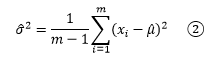a/(m - 1)肯定大于a/m，这使得②的结果稍大于①，m的值越小，①和②的差别越明显。随着样本数量的增加，取得极端值的机会也变大，①和②的差别也会越来越小。将样本的方差作为总体方差的点估计量，通常用s2表示。

值得一提的是，如果我们有m个样本，在计算这些样本的实际方差时，直接用①；如果是用这些样本估计总体的方差，应该使用②。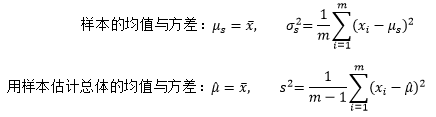# 估计总体的比例

很多人会在30分钟之内取得报告单，同样也有很多人要等更久。我们可以计算出样本中成功人数（30分钟之内拿到报告的人数）的比例，并用这个比例作为总体概率的点估计量：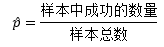到目前为止，点估计仍然很简单，所以经常有人吐槽：概率这么简单的玩意有啥值得研究的？

# 样本出现的概率

经过多年的统计分析，医院已经明确告知，每个患者都有50%的概率会在30分钟内拿到报告单。我们用p=50%表示总体中所有在30分钟之内拿到报告的人数的占比。如果把一个患者在30分钟之内拿到报告看作成功，用随机变量X表示m个样本中的成功数量，那么X符合参数为m和p的二项分布，X~B(m, p)，即成功次数符合试验次数和成功率的二项分布。保持试验次数m不变，二项分布近似于均值为mp、方差为mpq的正态分布（q = 1 - p）。

下面的代码画出了二项分布和其近似的正态分布：

import numpy as np
import matplotlib.pyplot as plt
from scipy import stats

fig = plt.figure(figsize=(10, 6))

p = 0.5 # 每次试验成功的概率
q = 1 - p # 每次试验失败的概率
m_list = [10, 15, 20] # 试验次数
c_list = ['r', 'g', 'b'] # 曲线颜色
m_max = max(m_list)

# 二项分布 X~B(m,p)
for i, m in enumerate(m_list):
ax = fig.add_subplot(3, 2, i * 2 + 1)
xs = np.arange(0, m + 1, 1) # 随机变量的取值
ys = stats.binom.pmf(xs, m, p) # 二项分布 X~B(m,p)
ax.vlines(xs, 0, ys, colors=c_list[i], label='m={}, p={}'.format(m, p))
ax.set_xticks(list(range(0, m_max + 1, 2))) # 重置x轴坐标
ax.set_xlabel('X')
ax.set_ylabel('pmf')
ax.set_title('X~B(m, p)')
ax.legend(loc='upper right')

# 保持二项分布试验的次数m不变，二项分布近似于均值为mp、方差为mp(1-p)的正态分布：
for i, m in enumerate(m_list):
ax = fig.add_subplot(3, 2, i * 2 + 2)
xs = np.arange(0, m + 1, 0.1) # 随机变量的取值
mu, sigma = m * p, (m * p * q) ** 0.5
ys = stats.norm.pdf(xs, mu, sigma)
ax.plot(xs, ys, c=c_list[i], label='m={}, p={}'.format(m, p))
ax.set_xticks(list(range(0, m_max + 1, 2)))  # 重置x轴坐标
ax.set_xlabel('X')
ax.set_ylabel('pdf')
ax.set_title('X~N(mp, mpq)')
ax.legend(loc='upper right')

plt.show()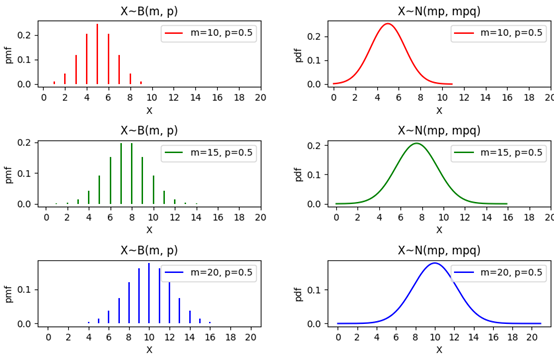某天来了20名患者，其中有12人在30分钟之内拿到了报告单（12个成功）。根据二项分布，这种情况出现的概率是：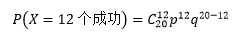100天过去，每天都有20名患者接受验血，xi人在30分钟内拿到了报告，每天的样本都对应一个概率：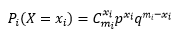上式中所有m­i的数量都是20，之所以用m­i表示，是为了强调虽然每天的样本数量一致，但样本本身是不同的。如果将这些概率也看成随机变量，那么这些变量也必然会符合某一个分布，只要弄清这个分布，就能回答产生某个样本的概率。既然可以通过样本知道样本中成功数量的占比，那么这个分布也就等同于“样本中成功数量的占比”的概率。比如第10天的样本中成功数量的占比是p10=45%，我们的目标是了解p10产生的概率有多大，即P(p10)=？换句话说，我们希望知道所有Pi(X=xi)构成的分布。

我们用ps表示某个特定样本中成功数量的占比，借助期望和方差来窥探ps的分布。一个明显的关系是，如果总体中有50%的人可以在30分钟内拿到报告，那么我们也同样期望在样本中看到这个比例，这也是我们能够用样本估计总体的基础。用随机变量X表示样本中成功的数量，ps = X/m：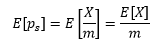我们已经知道X~B(m, p)，这里m是样本数量，p是每个样本成功的概率，是预先给出的。二项分布的期望是E[x] = mp，方差是Var(X)=mpq，q = 1 – p，因此：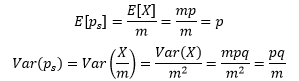E[ps]告诉我们，样本中成功数量的占比与整体中成功数量的占比一致；Var(p­s)告诉我们，m越大，p­s的方差越小，样本中成功数量的占比越近总体中成功数量的占比，用ps来估计p越可靠。既然二项分布X~B(m, p)可以由X~N(mp, mpq)来近似，那么p­s =X/m也可以由p­s~N(p, pq/m)来近似。对于本例来说，p=0.25，pq/m=0.0125：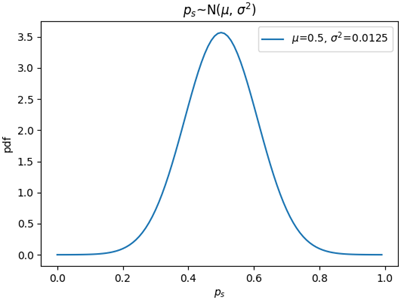值得注意的是，比例的分布刻画的是样本成功数占比（即X/m）的变化情况，而二项分布刻画的是特定数量的样本中成功数（即X）的变化情况。比例的取值范围是[0, 1]，因此在描述ps的分布时，随机变量的有效取值范围是[0, 1]。当m固定时，每个成功数占比都代表一个特定的样本，我们可以借用ps的分布计算从总体中抽样出某个固定数量样本的概率。

# 连续性修正

对于二项分布来说，保持试验次数n不变，二项分布近似于均值为np、方差为npq的正态分布。这里特别强调了“近似于”，是因为二项分布的随机变量是离散型的，而正态分布的随机变量是连续型，但是这又有什么关系呢？

这里先要了解一下离散型分布函数和连续型分布函数的特点。对于连续型分布来说，其分布函数是用密度函数的积分表示的：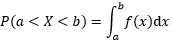对于积分来说，a~b的区间与是否包含a点或b点没什么关系，对于连续型随机变量的累积概率来说：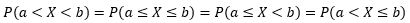但是上式对于离散型随机变量并不成立。下面是一个离散型分布函数，纵坐标的c.d.f是累积分布函数（cumulative distribution function）的缩写：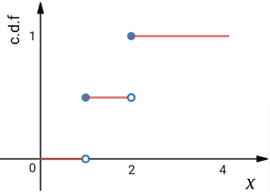上图向我们展示了P(X < 1) = 0，P(X ≤ 1) = 0.5。这意味着对于离散型随机变量来说，经常有P(x ≤ a) ≠ P(x < a)的情况（并不总是不等，这要看a的取值，对于上图来说，P(X < 1.5) = P(X ≤ 1.5)），而连续型随机变量总是有P(x≤a) = P(x<a)。

μ=50，σ2=25的正态分布X~N(μ, σ2)可以用来近似n=100，p=0.5的二项分布X~B(n, p)，下图是二者的分布函数（注意这里的曲线是分布函数，而不是密度函数）：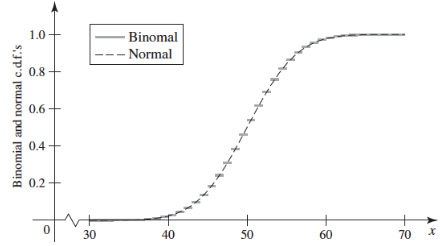可以看出，由于二项分布的离散型随机变量只能取到整数，因此它的分布函数是阶梯状的，而正态分布的曲线穿过了每个阶梯的中心点，将阶梯分成了两部分，左半部分离散分布大于连续分布，右半部分则相反：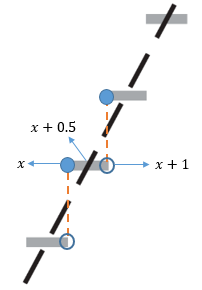分别用FB(x)=PB(X≤x)和Fn(x)=Pn(X≤x)表示二项分布和正态分布的分布函数，对于整数x来说，在[x, x+0.5)区间内，FB(x) > Fn(x)；在(x+0.5, x+1)区间内，FB(x) < Fn(x)；只有在中心点，才有FB(x) = Fn(x)。

现在问题来了，用正态分布去做近似的时候，如果直接用FN(x)去近似PB(X<x)，那么结果会偏大；如果用FN(x)去近似PB(X≤x)，则结果会偏小：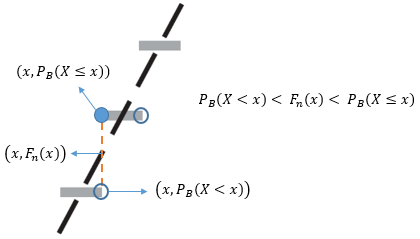时大时小并不是个好主意，我们想要的是一个一致的近似，要么总是大，要么总是小。一个办法是对于X的正态连续性修正为±0.5，即用FN(x+0.5)去近似PB(X < x)和PB(X ≤ x)，得到的结果不会偏小；或用FN(x-0.5)去近似PB(X < x)和PB(X ≤ x)，得到的结果不会偏大。这有点类似于用黎曼和计算积分时选用左矩形公式还是右矩形公式：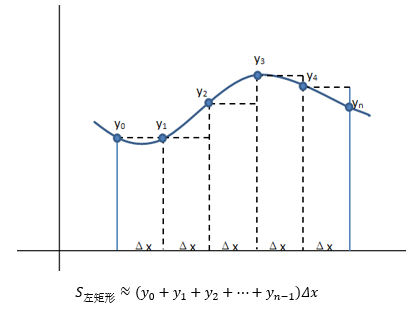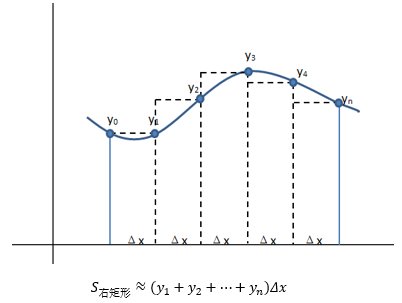回顾上一节的内容，我们计算出了样本占比p­s的正态分布近似，p­s的连续性修正为：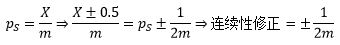借助连续性修正可以求得：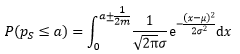出处：微信公众号 "我是8位的"

本文以学习、研究和分享为主，如需转载，请联系本人，标明作者和出处，非商业用途！

扫描二维码关注作者公众号“我是8位的”posted on 2020-02-10 12:25  我是8位的  阅读(1673)  评论(0编辑  收藏  举报